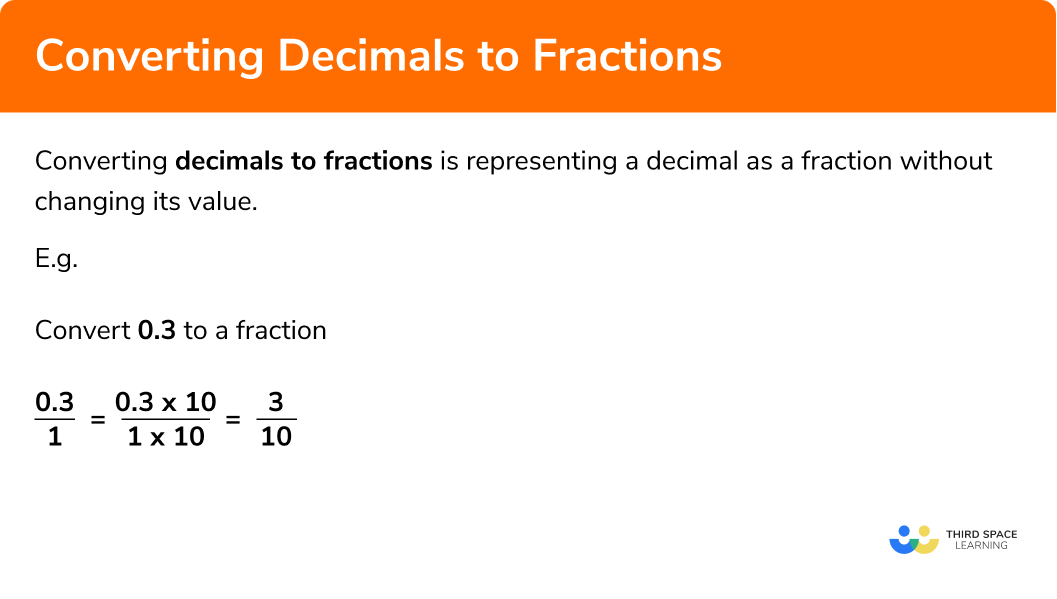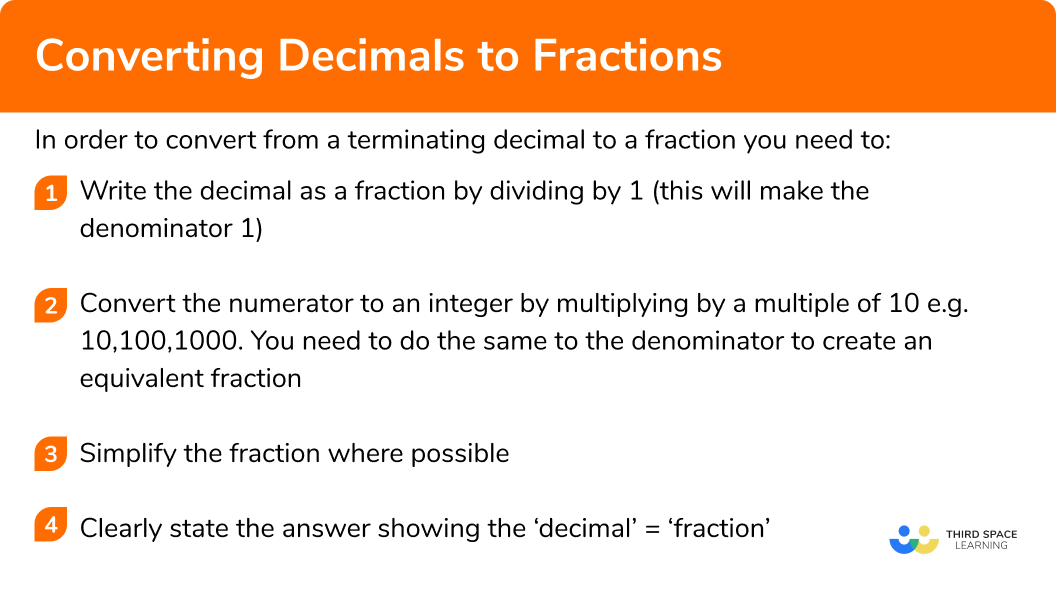GCSE Maths Number FDP Converting FDP

Decimals To Fractions

# Decimals To Fractions

Here we will learn about converting decimals to fractions.

There are also converting decimals to fractions worksheets based on Edexcel, AQA and OCR exam questions, along with further guidance on where to go next if you’re still stuck.

## What is decimals to fractions?

Converting decimals to fractions is representing a decimal as a fraction without changing its value.

E.g.

$0.25=\frac{1}{4}$
$0.125=\frac{1}{8}$
$0.2857142857…=\frac{2}{7}$
$3.2=3 \frac{1}{5}$

### What is converting decimals to fractions?Note: The decimals being converted in this page are terminating decimals, which means the decimal stops and does not have an infinite number of decimal places. We can also convert recurring decimals to fractions. Recurring decimals are decimals which have a repeating pattern that continually repeats without stopping.

## How to convert from decimals to fractions

In order to convert from a terminating decimal to a fraction you need to:

1. Write the decimal as a fraction by dividing by 1 (this will make the denominator 1)
2. Convert the numerator to an integer by multiplying by a multiple of 10, e.g. 10, 100, 1000. You need to do the same to the denominator to create an equivalent fraction
3. Simplify the fraction where possible
4. Clearly state the answer showing the ‘decimal’ = ‘fraction’

### Explain how to convert a terminating decimal to a fraction in 3 steps## Converting decimals to fractions examples

### Example 1: converting a simple decimal to a fraction (without simplifying)

Convert 0.3 to a fraction

1. Write the decimal as a fraction by dividing by 1

$0.3\div1$
$\frac{0.3}{1}$

2Convert the numerator to an integer (by multiplying by a multiple of 10). You need to do the same to the denominator to create an equivalent fraction

The lowest value in the number 0.3 is the 3 tenths.
This means if we multiply 0.3 by 10 we get the integer 3.

If you multiplied the numerator by 10 you would change the value of the whole fraction so you also need to multiply the denominator by 10. See below:

$\frac{0.3}{1}$
$\frac{0.3\times10}{1\times10}$
$\frac{3}{10}$

3Simplify the fraction if possible

\frac{3}{10} cannot be simplified as 3 and 10 do not have a common factor (which is not 1)

4Clearly state the answer showing the ‘decimal’ = ‘fraction’

$0.3=\frac{3}{10}$

### Example 2: converting a decimal to a fraction (with simplifying)

Convert 0.22 to a fraction

$0.22\div1$
$\frac{0.22}{1}$

The lowest value in the number 0.22 is the two hundredths.
This means if we multiply 0.22 by 100 we will end up with the integer value 22.

If you only multiplied the numerator by 100 you would change the value of the whole fraction so you also need to multiply the denominator by 100. See below:

$\frac{0.22}{1}$
$\frac{0.22\times100}{1\times100}$
$\frac{22}{100}$

\frac{22}{100} can be simplified by dividing the numerator and denominator by 2 (the highest common factor of 22 and 100)

$\frac{22\div2}{100\div2}$
$\frac{11}{50}$
$0.22=\frac{11}{50}$

### Example 3: converting a decimal to a fraction involving thousandths

Convert 0.385 to a fraction

$0.385\div1$
$\frac{0.385}{1}$

The lowest value in the number 0.385 is the 5 thousandths.
This means if we multiply 0.385 by 1000 we will end up with the integer value 385.

If you only multiplied the numerator by 1000 you would change the value of the whole fraction so you also need to multiply the denominator by 1000. See below:

$\frac{0.385}{1}$
$\frac{0.385\times1000}{1\times1000}$
$\frac{385}{1000}$

\frac{385}{1000} can be simplified by dividing the numerator and denominator by 5

$\frac{385\div5}{1000\div5}$
$\frac{77}{200}$

$0.385=\frac{77}{200}$

### Example 4: converting a number containing a decimal to a fraction

Convert 1.4 to a fraction

$1.4\div1$
$\frac{1.4}{1}$

The lowest value in the number 1.4 is the 4 tenths.
This means if we multiply 1.4 by 10 we will end up with the integer value 14.

If you only multiplied the numerator by 10 you would change the value of the whole fraction so you also need to multiply the denominator by 10. See below:

$\frac{1.4}{1}$
$\frac{1.4\times10}{1\times10}$
$\frac{14}{10}$

\frac{14}{10} can be simplified by dividing the numerator and denominator by 2

$\frac{14\div2}{10\div2}$
$\frac{7}{5}$

$1.4=\frac{7}{5}$

Note: This is an improper fraction, we could give this as a mixed number if required.

E.g.

$1.4=\frac{7}{5}=1\frac{2}{5}$

### Example 5: converting a number containing a decimal to a fraction

Convert 1.55 to a fraction

$1.55\div1$
$\frac{1.55}{1}$

The lowest value in the number 1.55 is the 5 hundredths.
This means if we multiply 1.55 by 100 we will end up with the integer value 155.

If you only multiplied the numerator by 100 you would change the value of the whole fraction so you also need to multiply the denominator by 100. See below:

$\frac{1.55}{1}$
$\frac{1.55\times100}{1\times100}$
$\frac{155}{100}$

\frac{155}{100} can be simplified by dividing the numerator and denominator by 5

$\frac{155\div5}{100\div5}$
$\frac{31}{50}$

$1.55=\frac{31}{50}$

### Example 6: converting a number containing a decimal to a fraction

Convert 20.0006 to a fraction

$20.0006\div1$
$\frac{20.0006}{1}$

The lowest value in the number 20.0006 is the 6 ‘ten thousandths’.
This means if we multiply 20.0006 by 10000 we will end up with the integer value 200006.

If you only multiplied the numerator by 10000 you would change the value of the whole fraction so you also need to multiply the denominator by 10000. See below:

$\frac{20.0006}{1}$
$\frac{20.0006\times10000}{1\times10000}$
$\frac{20006}{10000}$

\frac{20006}{10000} can be simplified by dividing the numerator and denominator by 2

$\frac{200006\div2}{100000\div2}$
$\frac{100003}{5000}$

$20.0006=\frac{100003}{5000}$

### Common misconceptions

• Multiplying by an incorrect multiple of 10

You must multiply by a multiple of 10 that results in the numerator being an integer (a whole number). Use your knowledge of place value to help decide which multiple of 10 to multiply by.

E.g.
0.003 × 10 = 0.03 which is not an integer

• Simplifying the fraction

The question may say “give your answer in the simplest form”. Always take a moment to see if the fraction can be simplified.

• Not multiplying the denominator by the same as the numerator

When you multiply the numerator by a multiple of 10 you must do the same for the denominator otherwise you are changing the value of the fraction.

Decimals to fractions is part of our series of lessons to support revision on comparing fractions, decimals and percentages. You may find it helpful to start with the main comparing fractions, decimals and percentages lesson for a summary of what to expect, or use the step by step guides below for further detail on individual topics. Other lessons in this series include:

### Practice converting decimals to fractions questions

1. Which is the correct conversion of 0.1 to a fraction in its simplest form?

\frac{10}{100}\frac{1}{10}\frac{0.1}{1}\frac{0.01}{100}0.1 written as a fraction is \frac{10}{100} . We can simplify this by dividing both the numerator and denominator by 10 .

2. Which is the correct conversion of 0.4 to a fraction in its simplest form?

\frac{40}{100}\frac{4}{10}\frac{2}{5}\frac{0.4}{1}0.4 written as a fraction is \frac{40}{100} . We can simplify this by dividing both the numerator and denominator by 20 .

3. Which is the correct conversion of 1.1 to a fraction in its simplest form?

\frac{1.1}{1}\frac{11}{10}\frac{110}{100}\frac{1}{10}We can convert 1.1 to a fraction by writing it over 1 and multiplying the numerator and denominator by 10 .

4. Which is the correct conversion of 0.006 to a fraction in its simplest form?

\frac{0.006}{1}\frac{6}{1}\frac{6}{1000}\frac{3}{500}0.006 written as a fraction is \frac{6}{1000} . We can simplify this by dividing both the numerator and denominator by 2 .

5. Which is the correct conversion of 30.05 to a fraction in its simplest form?

\frac{601}{20}\frac{1}{20}30 \frac{1}{20}\frac{3005}{1000}We can convert 30.05 to a fraction by writing it over 1 and multiplying the numerator and denominator by 100 to give \frac{3005}{1000} . We can simplify this by dividing both the numerator and denominator by 5 .

6. Which of the below is not the fractional equivalent of 0.12 ?

\frac{84}{700}\frac{12}{100}\frac{6}{50}\frac{12}{10}\frac{12}{10} is equivalent to 1.2 not 0.12 .

### Converting decimals to fractions GCSE questions

1. Convert each of the following decimals to fractions. All answers must be given in their simplest form

a) 0.7

b) 0.75

c) 0.07

d) 0.007

e) 7.7

(5 marks)

\frac{7}{10}

(1)

\frac{3}{4}

(1)

\frac{7}{100}

(1)

\frac{7}{1000}

(1)

\frac{77}{10}

(1)

2. Convert each of the following decimals to fractions in their simplest form

a) 0.34

b) 1.12

c) 1.72

(6 marks)

1 mark for any correct fraction given which is not in its simplest form

a) \frac{34}{100}

(1)

OR

\frac{17}{50}

(2)

b) \frac{112}{100}

(1)

OR

\frac{28}{25}

(2)

c) \frac{172}{100}

(1)

OR

\frac{43}{25}

(2)

3. Match each decimal to the correct fraction below

\frac{3}{5},    \frac{1}{4} ,    \frac{7}{5},    \frac{26}{5},   \frac{1}{100} Q3

a) 0.25

b) 1.4

c) 0.6

d) 0.01

e) 5.2

(5 marks)

0.25=\frac{1}{4}

(1)

1.4=\frac{7}{5}

(1)

0.6=\frac{3}{5}

(1)

0.01=\frac{1}{100}

(1)

5.2=\frac{26}{5}

(1)

4. Show 0.888 as a fraction in its simplest form

(2 marks)

1 mark for any correct fraction given which is not in its simplest form

\frac{888}{1000}

(1)

\frac{111}{125}

(1)

## Learning checklist

You have now learned how to:

• Convert a decimal to a fraction
• Give a fraction in its simplest form

## Still stuck?

Prepare your KS4 students for maths GCSEs success with Third Space Learning. Weekly online one to one GCSE maths revision lessons delivered by expert maths tutors.

Find out more about our GCSE maths tuition programme.